﻿ Parabolas

#Parabolas

DEFINITION parabola
A parabola is the set of points in a plane that are the same distance from a fixed point (called the focus), and a fixed line (called the directrix).

To explore this definition, investigate the diagram below.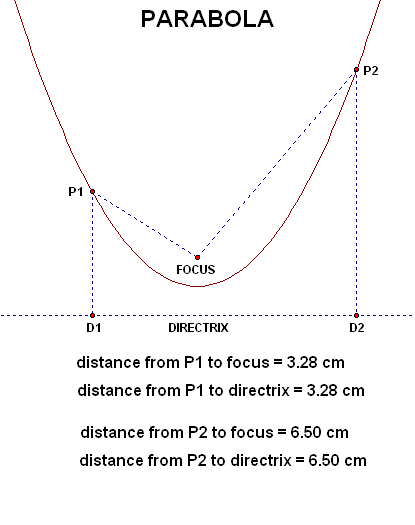Pick any point on the parabola (say, $\,\text{P}1\,$). Travel to the focus, and record this distance ($3.28 \text{ cm}$). Go back to $\,\text{P}1\,,$ and now travel to the directrix ($3.28 \text{ cm}$). (Note that the distance from a point to a line is the shortest distance from the point to the line, which is measured along the perpendicular.) These two distances are the same!

Pick a different point on the parabola (say, $\,\text{P}2\,$). Travel to the focus, and record this distance ($6.50 \text{ cm}$). Go back to $\,\text{P}2\,,$ and now travel to the directrix ($6.50 \text{ cm}$). Again, these two distances are the same!

No matter what point you choose on a parabola, its distances to the focus and the directrix will be equal.

You can play with parabolas here. (Drag the focus and directrix around; watch the parabola change!)

Parabolas have some beautiful geometric properties that make them very important in real-life applications.

REFLECTING PROPERTY OF PARABOLAS
Rays emanating from the focus will always be reflected perpendicular to the directrix.

To explore this property, investigate the diagram below.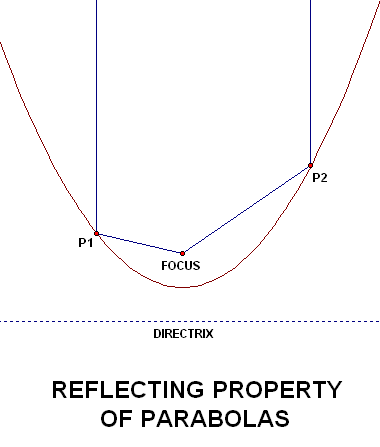Place a light at the focus. The beams will go out and hit the parabola. They will always be reflected perpendicular to the directrix, as shown.

This ability of parabolas to generate straight, focused beams of light makes them valuable in applications as varied as laser surgery and the Hollywood beams of light!

This same geometric property also allows parabolas to act as collectors:

COLLECTING PROPERTY OF PARABOLAS
Rays entering the parabola perpendicular to the directrix are always reflected so that they pass through the focus.

Think about the satellite dish on the outside of a house. Beams enter the dish at all possible angles, but those that come in perpendicular to the directrix are all focused on a single point, where a device collects the signal, amplifies it, and sends it into the house.

Thus, the name focus is appropriate for this special point!

VERTEX OF A PARABOLA
The vertex of a parabola is the point that is exactly halfway between the focus and the directrix. It is the point where the parabola turns (i.e., changes direction).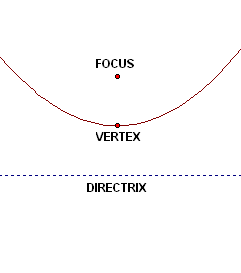The distance between the focus and the vertex affects the shape of the parabola, as shown below.

As the focus moves farther away from the vertex, the parabola gets wider (flatter). As the focus moves closer to the vertex, the parabola gets narrower (steeper).

In any orientation of a parabola, the focus is always inside the parabola.

When the focus is above the vertex, then the parabola holds water (i.e., is concave up). When the focus is below the vertex, the parabola sheds water (i.e., is concave down).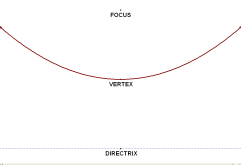wide parabola; focus far from vertex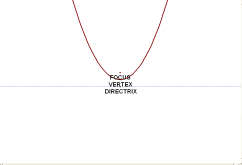narrow parabola; focus close to vertex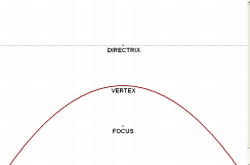concave down